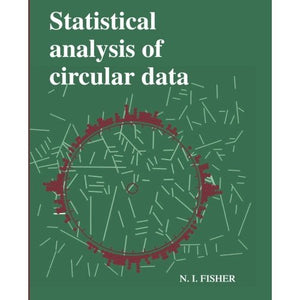># Statistical Analysis of Circular Data

• £21.99
• Save £14

N. I. Fisher
Cambridge University Press
Edition: New Ed, 10/12/1995
EAN 9780521568906, ISBN10: 0521568900

Paperback, 296 pages, 24.7 x 17.4 x 1.7 cm
Language: English

Data measured as angles or two-dimensional orientations are found almost everywhere in science. They commonly arise in biology, geography, geophysics, medicine, meteorology and oceanography, and many other areas. Examples of such data include departure directions of birds from release points, fracture plane orientations, the directional movement of animals after stimulation, wind and ocean current directions, and biorhythms. Statistical methods for handling such data have developed rapidly in the last twenty years, particularly data display, correlation, regression and analysis of tempered or spatially structured data. Further, some of the exciting modern developments in general statistical methodology, particularly nonparametric smoothing methods and bootstrap-based methods, have contributed significantly to relatively intractable data analysis problems. This book provides a unified and up-to-date account of techniques for handling circular data.

Preface
1. The purpose of the book
2. Survey of contents
3. How to use the book
4. Notation, terminology and conventions
5. Acknowledgements
Part I. Introduction
Part II. Descriptive Methods
2.1. Introduction
2.2. Data display
2.3. Simple summary quantities
2.4. Modifications for axial data
Part III. Models
3.1. Introduction
3.2. Notation
trigonometric moments
3.3. Probability distributions on the circle
Part IV. Analysis of a Single Sample of Data
4.1. Introduction
4.2. Exploratory analysis
4.3. Testing a sample of unit vectors for uniformity
4.4. Nonparametric methods for unimodal data
4.5. Statistical analysis of a random sample of unit vectors from a von Mises distribution
4.6. Statistical analysis of a random sample of unit vectors from a multimodal distribution
4.7. Other topics
Part V. Analysis of Two or More Samples, and of Other Experimental Layouts
5.1. Introduction
5.2. Exploratory analysis
5.3. Nonparametric methods for analysing two or more samples of unimodal data
5.4. Analysis of two or more samples from von Mises distributions
5.5. Analysis of data from more complicated experimental designs
Part VI. Correlation and Regression
6.1. Introduction
6.2. Linear-circular association and circular-linear association
6.3. Circular-circular association
6.4. Regression models for a circular response variable
Part VII. Analysis of Data with Temporal or Spatial Structure
7.1. Introduction
7.2. Analysis of temporal data
7.3. Spatial analysis
Part VIII. Some Modern Statistical Techniques for Testing and Estimation
8.1. Introduction
8.2. Bootstrap methods for confidence intervals and hypothesis tests
general description
8.3. Bootstrap methods for circular data
confidence regions for the mean direction
8.4. Bootstrap methods for circular data
hypothesis tests for mean directions
8.5. Randomisation, or permutation, tests
Appendix A. Tables
Appendix B. Data sets
References
Index.